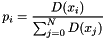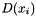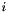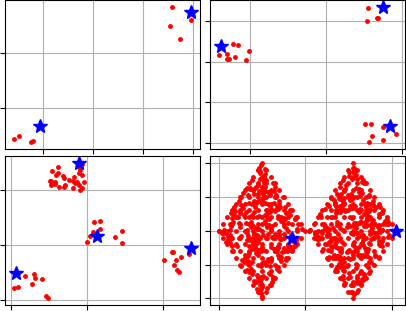pyclustering.cluster.center_initializer.kmeans_plusplus_initializer Class Reference

K-Means++ is an algorithm for choosing the initial centers for algorithms like K-Means or X-Means. More...

## Public Member Functions

def __init__ (self, data, amount_centers, amount_candidates=None)
Creates K-Means++ center initializer instance. More...

def initialize (self, kwargs)
Calculates initial centers using K-Means++ method. More...

## Static Public Attributes

string FARTHEST_CENTER_CANDIDATE = "farthest"
Constant denotes that only points with highest probabilities should be considered as centers. More...

## Detailed Description

K-Means++ is an algorithm for choosing the initial centers for algorithms like K-Means or X-Means.

K-Means++ algorithm guarantees an approximation ratio O(log k). Clustering results are depends on initial centers in case of K-Means algorithm and even in case of X-Means. This method is used to find out optimal initial centers.

Algorithm can be divided into three steps. The first center is chosen from input data randomly with uniform distribution at the first step. At the second, probability to being center is calculated for each point:whereis a distance from pointto the closest center. Using this probabilities next center is chosen. The last step is repeated until required amount of centers is initialized.

Pyclustering implementation of the algorithm provides feature to consider several candidates on the second step, for example:

amount_centers = 4;
amount_candidates = 3;
initializer = kmeans_plusplus_initializer(sample, amount_centers, amount_candidates);

If the farthest points should be used as centers then special constant 'FARTHEST_CENTER_CANDIDATE' should be used for that purpose, for example:

amount_centers = 4;
amount_candidates = kmeans_plusplus_initializer.FARTHEST_CENTER_CANDIDATE;
initializer = kmeans_plusplus_initializer(sample, amount_centers, amount_candidates);

There is an example of initial centers that were calculated by the K-Means++ method:Code example where initial centers are prepared for K-Means algorithm:

from pyclustering.cluster.center_initializer import kmeans_plusplus_initializer
from pyclustering.cluster.kmeans import kmeans
from pyclustering.cluster import cluster_visualizer
from pyclustering.samples.definitions import SIMPLE_SAMPLES
# Read data 'SampleSimple3' from Simple Sample collection.
# Calculate initial centers using K-Means++ method.
centers = kmeans_plusplus_initializer(sample, 4, kmeans_plusplus_initializer.FARTHEST_CENTER_CANDIDATE).initialize()
# Display initial centers.
visualizer = cluster_visualizer()
visualizer.append_cluster(sample)
visualizer.append_cluster(centers, marker='*', markersize=10)
visualizer.show()
# Perform cluster analysis using K-Means algorithm with initial centers.
kmeans_instance = kmeans(sample, centers)
# Run clustering process and obtain result.
kmeans_instance.process()
clusters = kmeans_instance.get_clusters()

Definition at line 104 of file center_initializer.py.

## ◆ __init__()

 def pyclustering.cluster.center_initializer.kmeans_plusplus_initializer.__init__ ( self, data, amount_centers, amount_candidates = None )

Creates K-Means++ center initializer instance.

Parameters
 [in] data (array_like): List of points where each point is represented by list of coordinates. [in] amount_centers (uint): Amount of centers that should be initialized. [in] amount_candidates (uint): Amount of candidates that is considered as a center, if the farthest points (with the highest probability) should be considered as centers then special constant should be used 'FARTHEST_CENTER_CANDIDATE'.
FARTHEST_CENTER_CANDIDATE

Definition at line 173 of file center_initializer.py.

## ◆ initialize()

 def pyclustering.cluster.center_initializer.kmeans_plusplus_initializer.initialize ( self, kwargs )

Calculates initial centers using K-Means++ method.

Parameters
 [in] **kwargs Arbitrary keyword arguments (available arguments: 'return_index').

Keyword Args:

• return_index (bool): If True then returns indexes of points from input data instead of points itself.
Returns
(list) List of initialized initial centers. If argument 'return_index' is False then returns list of points. If argument 'return_index' is True then returns list of indexes.

Definition at line 334 of file center_initializer.py.

## ◆ FARTHEST_CENTER_CANDIDATE

 string pyclustering.cluster.center_initializer.kmeans_plusplus_initializer.FARTHEST_CENTER_CANDIDATE = "farthest"
static

Constant denotes that only points with highest probabilities should be considered as centers.

Definition at line 170 of file center_initializer.py.

The documentation for this class was generated from the following file: